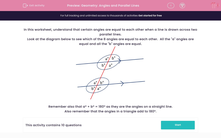# Geometry: Angles and Parallel Lines

In this worksheet, students work out the values of angles in diagrams with parallel lines.This content is premium and exclusive to EdPlace subscribers.Key stage:  KS 2

Curriculum topic:   Maths and Numerical Reasoning

Curriculum subtopic:   2D Shapes: Circles, Angles and Bearings

Difficulty level:#### Worksheet Overview

In this worksheet, understand that certain angles are equal to each other when a line is drawn across two parallel lines.

Look at the diagram below to see which of the 8 angles are equal to each other.  All the "a" angles are equal and all the "b" angles are equal.Remember also that aº + bº = 180º as they are the angles on a straight line.

Also remember that the angles in a triangle add to 180º.

### What is EdPlace?

We're your National Curriculum aligned online education content provider helping each child succeed in English, maths and science from year 1 to GCSE. With an EdPlace account you’ll be able to track and measure progress, helping each child achieve their best. We build confidence and attainment by personalising each child’s learning at a level that suits them.

Get started